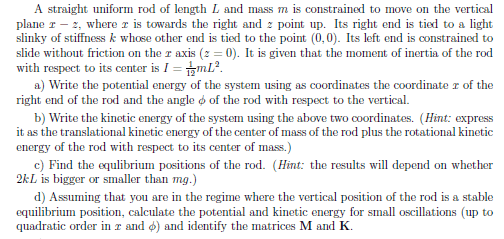# Rod-spring system

## Homework StatementI'm really looking for a verification on parts a) and b), but I'll add what I did with part c) without going to into too much detail. I'm posting this question mainly due to part d). I feel that I have every part before this right, but I'm not getting any symmetric matrices.

## Homework Equations

##T=\frac{1}{2}mv^2##

## The Attempt at a Solution

a)##U=\dfrac{mgL}{2}\cos{\phi}+\dfrac{k}{2}(x^2+L^2(\cos{\phi})^2)##
b)##x_{cm}=x-\dfrac{L}{2}\sin(\phi)##, ##\dot{x_cm}=1-\dfrac{L}{2}\dot{\phi}\cos{\phi}##
##z_{cm}=\dfrac{L}{2}\cos(\phi)##, ##\dot{z_cm}=-\dfrac{\dot{\phi}}{2}L\sin(\phi)##
##T=\dfrac{mL^2\dot{\phi}^2}{6}+\dfrac{m}{2}(1-L\dot{\phi}\cos{\phi})##
c) I know for part c) we can just use the Lagrangian of the system, then find the Euler-Lagrange and let the acceleration in the ##\phi## and ##x## directions be zero.

Last edited: